Solving Linear differential equations - Statement given

Chapter 9 Class 12 Differential Equations
Concept wise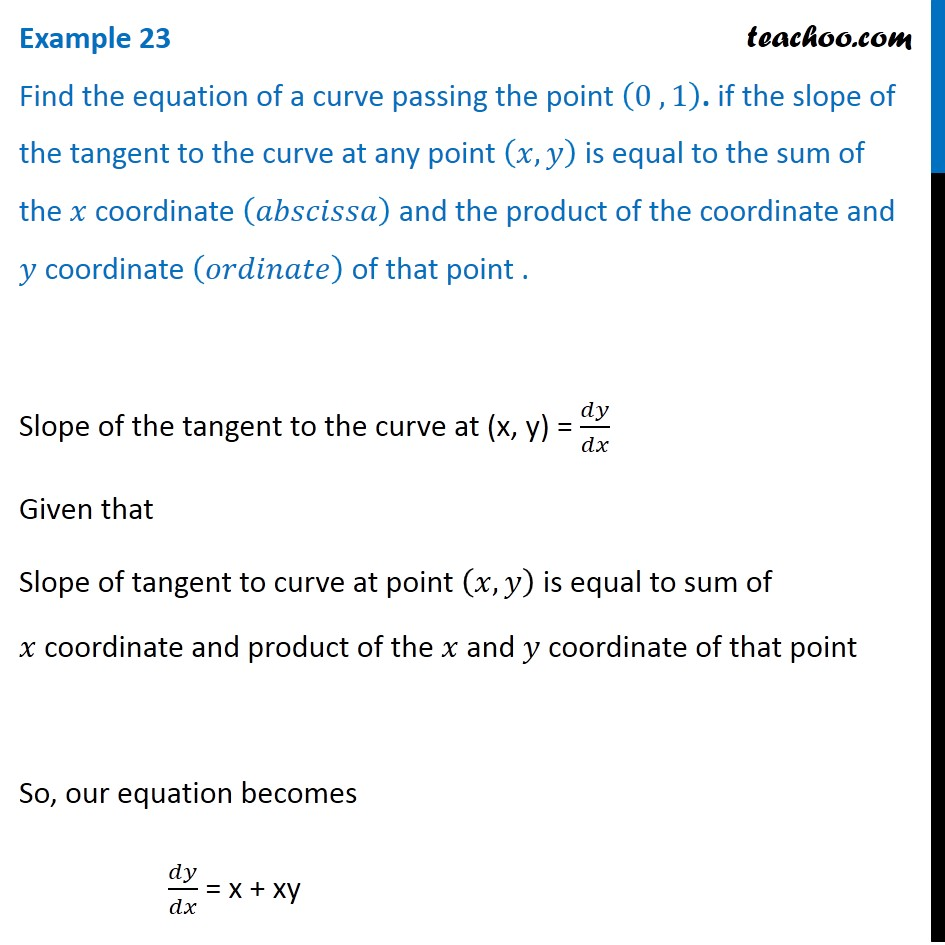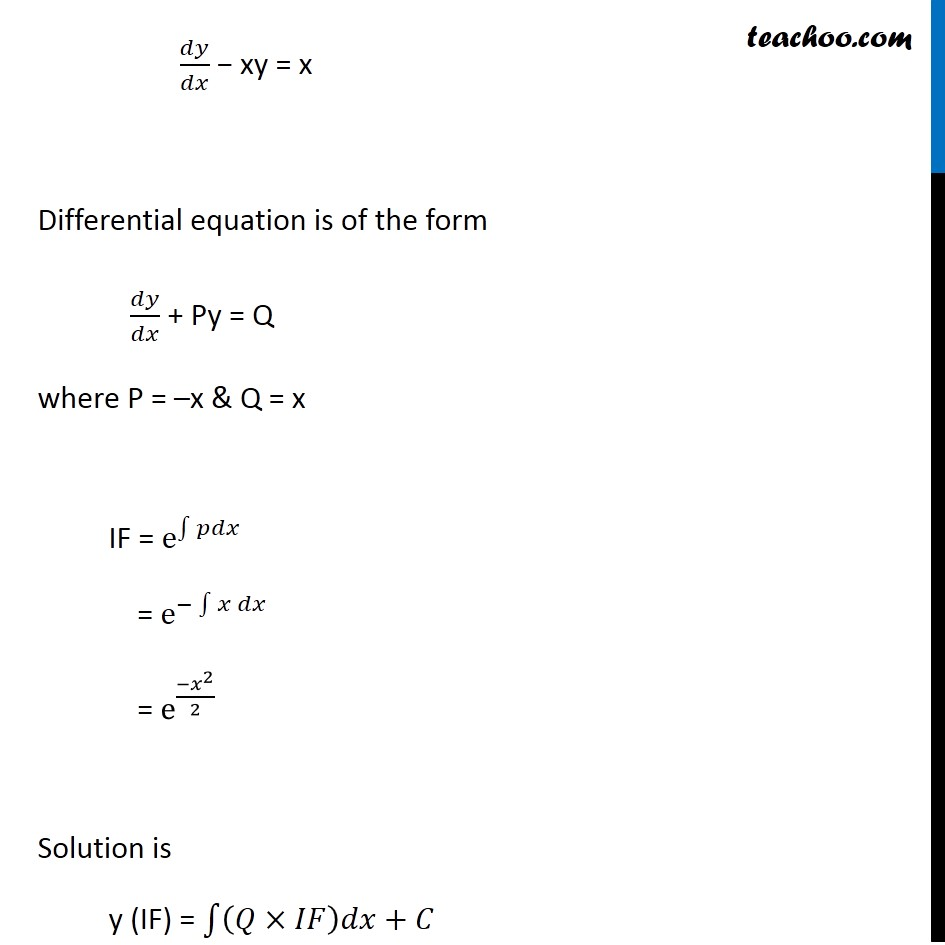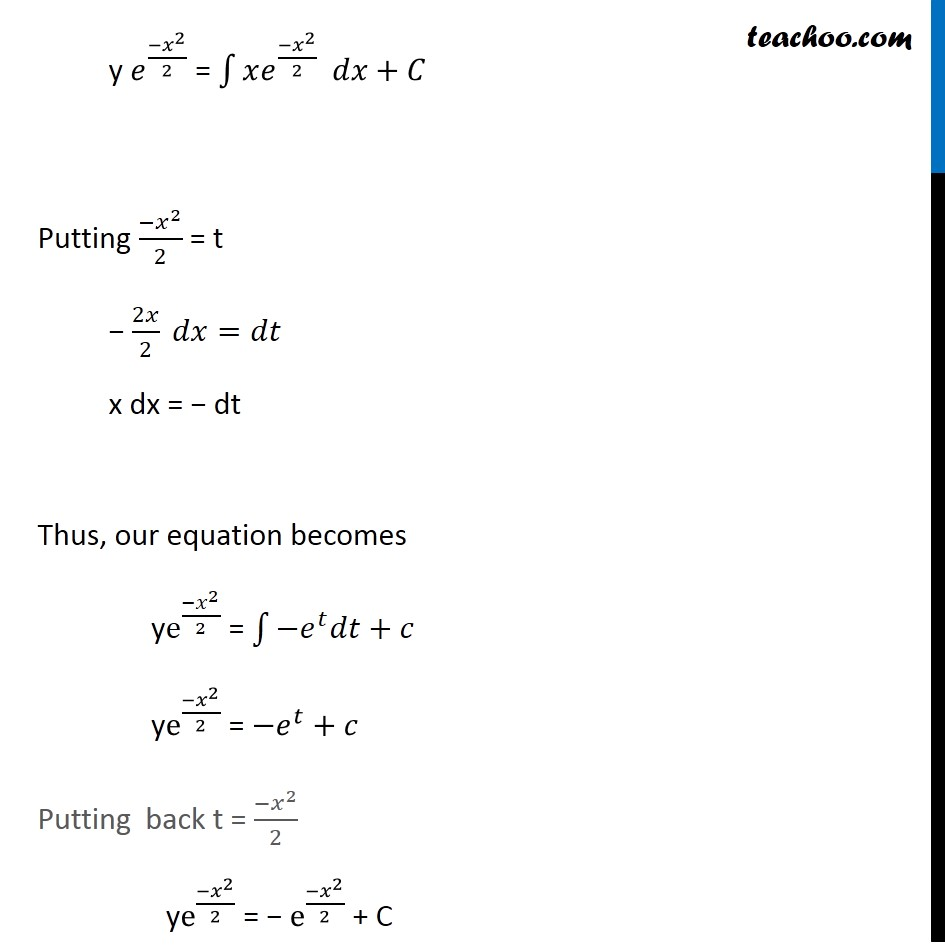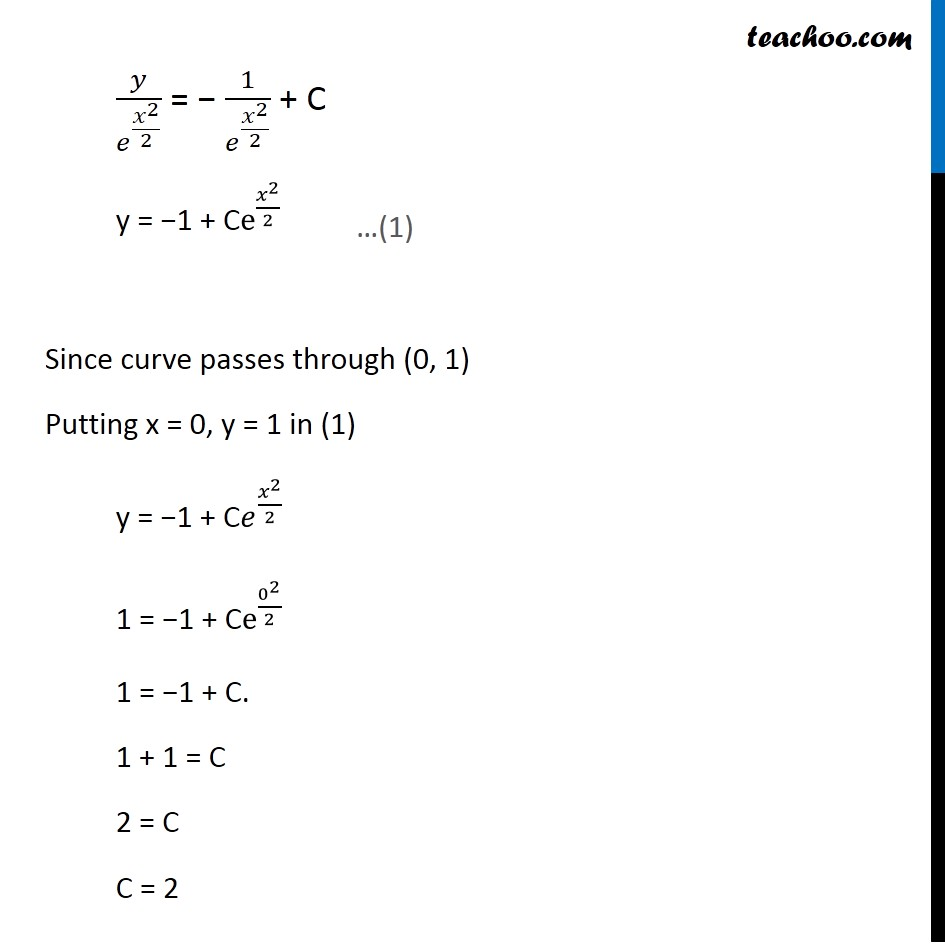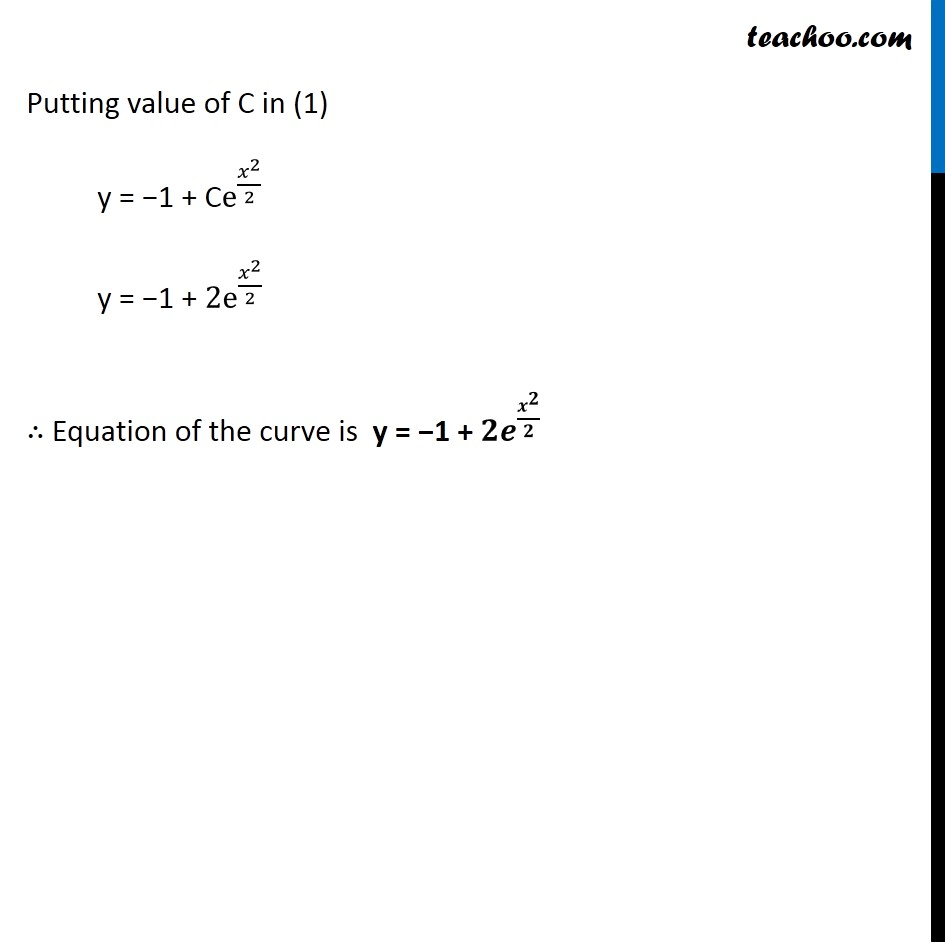Solve all your doubts with Teachoo Black (new monthly pack available now!)

### Transcript

Example 23 Find the equation of a curve passing the point (0 , 1). if the slope of the tangent to the curve at any point (𝑥, 𝑦) is equal to the sum of the 𝑥 coordinate (𝑎𝑏𝑠𝑐𝑖𝑠𝑠𝑎) and the product of the coordinate and 𝑦 coordinate (𝑜𝑟𝑑𝑖𝑛𝑎𝑡𝑒) of that point . Slope of the tangent to the curve at (x, y) = 𝑑𝑦/𝑑𝑥 Given that Slope of tangent to curve at point (𝑥, 𝑦) is equal to sum of 𝑥 coordinate and product of the 𝑥 and 𝑦 coordinate of that point So, our equation becomes 𝑑𝑦/𝑑𝑥 = x + xy 𝑑𝑦/𝑑𝑥 − xy = x Differential equation is of the form 𝑑𝑦/𝑑𝑥 + Py = Q where P = –x & Q = x IF = e^∫1▒𝑝𝑑𝑥 = e^(−∫1▒〖𝑥 𝑑𝑥〗) = e^(〖−𝑥〗^2/2) Solution is y (IF) = ∫1▒〖(𝑄×𝐼𝐹)𝑑𝑥+𝐶〗 y 𝑒^((−𝑥^2)/2) = ∫1▒〖𝑥𝑒^(〖−𝑥〗^2/2) 𝑑𝑥+𝐶〗 Putting (−𝑥^2)/2 = t − 2𝑥/2 𝑑𝑥=𝑑𝑡 x dx = − dt y 𝑒^((−𝑥^2)/2) = ∫1▒〖𝑥𝑒^(〖−𝑥〗^2/2) 𝑑𝑥+𝐶〗 Putting (−𝑥^2)/2 = t − 2𝑥/2 𝑑𝑥=𝑑𝑡 x dx = − dt Thus, our equation becomes ye^((−𝑥^2)/2) = ∫1▒〖−𝑒^𝑡 𝑑𝑡+𝑐〗 ye^((−𝑥^2)/2) = −𝑒^𝑡+𝑐 Putting back t = (−𝑥^2)/2 ye^((−𝑥^2)/2) = − e^((−𝑥^2)/2) + C 𝑦/𝑒^(𝑥^2/2) = − 1/𝑒^(𝑥^2/2) + C y = −1 + Ce^(𝑥^2/2) Since curve passes through (0, 1) Putting x = 0, y = 1 in (1) y = −1 + C𝑒^(𝑥^2/2) 1 = −1 + Ce^(0^2/2) 1 = −1 + C. 1 + 1 = C 2 = C C = 2 …(1) Putting value of C in (1) y = −1 + Ce^(𝑥^2/2) y = −1 + 2e^(𝑥^2/2) ∴ Equation of the curve is y = −1 + 𝟐𝒆^(𝒙^𝟐/𝟐)